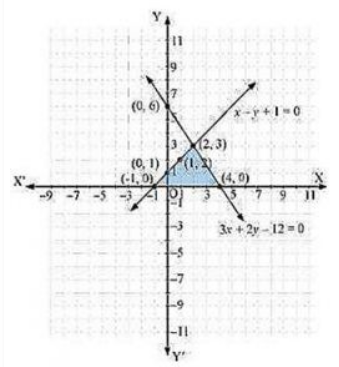# Draw the graphs of the equations x - y + 1 = 0 and 3x + 2y - 12 = 0. Determine the coordinates of the vertices of the triangle formed by these lines and the x-axis, and shade the triangular region.

Given, the equations for graphs are x – y + 1 = 0 and 3x + 2y – 12 = 0.

For, x – y + 1 = 0 or x = 1+y

 x 0 1 2 y 1 2 3

For, 3x + 2y – 12 = 0 or x = (12-2y)/3

 x 4 2 0 y 0 3 6

The graphical represnetaion of the equations areFrom the figure, we notice that these lines are intersecting each other at point (2, 3) and x-axis at (−1, 0) and (4, 0). Therefore, the vertices of the triangle are (2, 3), (−1, 0), and (4, 0).